Bracket Checker : Stack « Collections Data Structure « Java

Bracket Checker

```
import java.io.IOException;

public class BracketChecker {
private String input;

public BracketChecker(String in) {
input = in;
}

public void check() {
int stackSize = input.length();
Stack theStack = new Stack(stackSize);

for (int j = 0; j < input.length(); j++)
{
char ch = input.charAt(j);
switch (ch) {
case '{': // opening symbols
case '[':
case '(':
theStack.push(ch); // push them
break;

case '}': // closing symbols
case ']':
case ')':
if (!theStack.isEmpty()) // if stack not empty,
{
char chx = theStack.pop(); // pop and check
if ((ch == '}' && chx != '{') || (ch == ']' && chx != '[')
|| (ch == ')' && chx != '('))
System.out.println("Error: " + ch + " at " + j);
} else
// prematurely empty
System.out.println("Error: " + ch + " at " + j);
break;
default: // no action on other characters
break;
}
}
if (!theStack.isEmpty())
System.out.println("Error: missing right delimiter");
}

public static void main(String[] args) throws IOException {
String input = "{Java [Source] (and) {[(Support)]}}";
BracketChecker theChecker = new BracketChecker(input);
theChecker.check();
}

}

class Stack {
private int maxSize;

private char[] stackArray;

private int top;

public Stack(int max) {
maxSize = max;
stackArray = new char[maxSize];
top = -1;
}

public void push(char j) {
stackArray[++top] = j;
}

public char pop() {
return stackArray[top--];
}

public char peek() {
return stackArray[top];
}

public boolean isEmpty() {
return (top == -1);
}
}

```

Related examples in the same category

 1 Demonstration of Stack Class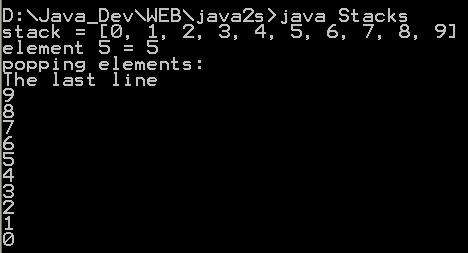2 Stack in java.util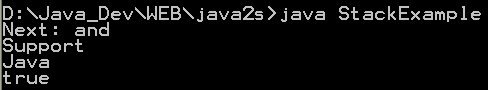3 Stack data structure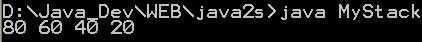4 String Reverser Through Stack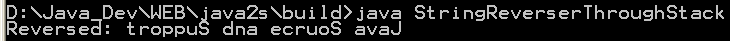5 Link stack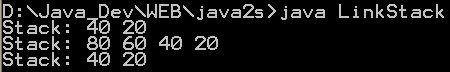6 Triangular numbers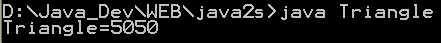7 Triangular numbers with stack replaces recursion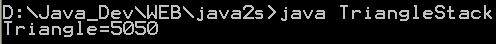8 Show String Reversals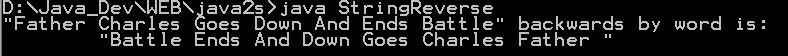9 Generic stack demo with annotation 10 Character Stack 11 A faster, smaller stack implementation. 12 Growable Object stack with type specific access methods 13 Growable int stack with type specific access methods 14 Stack for boolean values 15 extends ArrayList to create Stack 16 Growable String stack with type specific access methods. 17 A simple integer based stack. 18 A very simple unsynchronized stack. This one is faster than the java.util-Version. 19 Pop an empty stack ntry times and catch the resulting exception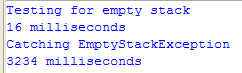20 Object Stack 21 Fast stack